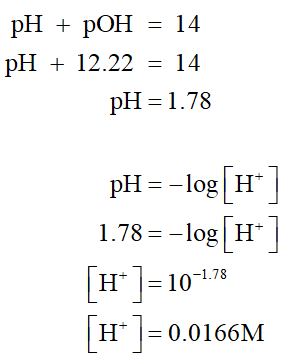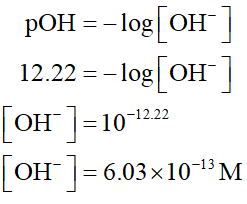# Given the pOH is 12.22a. what is [H+]b. what os [OH-]c. is it acidic or basic?

Question
1 views

Given the pOH is 12.22

a. what is [H+]

b. what os [OH-]

c. is it acidic or basic?

check_circle

Step 1

a.

The relationship between the hydronium ion concentration and the hydroxide ion concentration is given by the equation as follows,

pH + pOH = 14.

From given pOH, the pH is first calculated. Then [H+] concentration is determined as shown below,Step 2

b.

The  value is negative log of [OH-]-Hydroxide ion concentration....

### Want to see the full answer?

See Solution

#### Want to see this answer and more?

Solutions are written by subject experts who are available 24/7. Questions are typically answered within 1 hour.*

See Solution
*Response times may vary by subject and question.
Tagged in